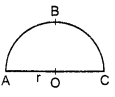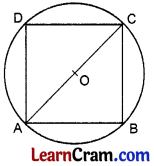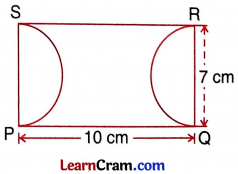# DAV Class 7 Maths Chapter 11 Worksheet 6 Solutions

The DAV Maths Class 7 Solutions and DAV Class 7 Maths Chapter 11 Worksheet 6 Solutions of Perimeter and Area offer comprehensive answers to textbook questions.

## DAV Class 7 Maths Ch 11 WS 6 Solutions

Question 1.
Find the area of a circle of diameter 8.4 m.
Diameter = 8.4 m
radius = $$\frac{8.4}{2}$$ = 4.2 m
Area of circle = πr2
= $$\frac{22}{7}$$ × 4.2 × 4.2
= 22 × 0.6 × 4.2 = 55.44 m2
Hence the required area = 55.44 m2.

Question 2.
The area of a circular lawn is 154 m2. Find its circumference.
Area = 154 m2
πr2 = 154
$$\frac{22}{7}$$ × r2 = 154
r2 = $$\frac{154 \times 7}{22}$$
r2 = 7 × 7
r = 7m
Circumference = 2πr = 2 × $$\frac{22}{7}$$ × 7 = 44 m
Hence the required circumference = 44 m.Question 3.
A circular plot is of radius 77 m. Find its value at ₹ 57 per square meter.
Area of the circular plot = πr2
= $$\frac{22}{7}$$ × 77 × 77
= 22 × 11 × 77
= 18634 m2
Value of the plot = ₹ 57 × 18634 = ₹ 10,62,138
Hence the value of the plot = ₹ 10,62,138

Question 4.
The diameters of two silver discs are in the ratio 2:3. What will be the ratio of their areas?
Let the diameters of the two silver discs be 2x cm and 3x cm respectively.
Their radii are x cm and $$\frac{3x}{2}$$ cm
If A1 and A2 be their respective areas
∴ $$\frac{A_1}{A_2}=\frac{\pi(x)^2}{\pi(3 x / 2)^2}$$
⇒ $$\frac{A_1}{A_2}=\frac{x^2}{\frac{9}{4} x^2}=\frac{4}{9}$$
Hence the required ratio is 4 : 9.

Question 5.
If the perimeter of a semi-circular park is 72 m,find its area and radius.AC + ABC = 72 m
2r + πr = 72
r (2 + π) = 72
r (2 + $$\frac{22}{7}$$) = 72
r × $$\frac{36}{7}$$ = 72
r = 72 × $$\frac{7}{36}$$ = 14 m

Area of the park = $$\frac{1}{2}$$πr2
= $$\frac{1}{2} \times \frac{22}{7}$$ × 14 × 14
= $$\frac{1}{2}$$ × 616 m2
= 308 m2
Hence the required area is 308 m2 and the radius = 14 m.

Question 6.
If a wire of 440 m length is moulded in the form of a circle and a square turn-by-turn, find the ratio of the area of the circle to that of square.
Answer:If it is moulded into a circle,
then the circumference C = 2πr
440 = 2 × $$\frac{22}{7}$$ × r
r = $$\frac{440 \times 7}{2 \times 22}$$ = 70 cm
Area of the circle = πr2
= $$\frac{22}{7}$$ × 70 × 70 = 15400 cm2
If the wire is moulded into a square,
then its perimeter = 440 m
4 × Side = 440 m
Side = $$\frac{440}{4}$$ = 110 m
Area of the square = (side)2
= 110 × 110 = 12100 m2 .
∴ $$\frac{\text { Area of the circle }}{\text { Area of the square }}=\frac{15400}{12100}=\frac{14}{11}$$
Hence the required ratio is 14 :11.

Question 7.
Find the area of a square that can be inscribed in a circle of radius 8 cm.
Let ABCD be a square inscribed in a circle of radius ‘r’.∴ AC = 2 r
In right ΔABC,
AB2 + BC2 = (2r)2 (By Pythagoras theorem)
AB2 + AB2 = 4r2
2AB2 = 4r2
AB2 = 2r2
∴ AB = √2r = √2 × 8 = 8√2 cm

Area of the square = (side)2
= (8√2)2 = 64 × 2 = 128 cm2
Hence the required area = 128 cm2.

Question 8.
The diameter of a circular park is 24 m. A 3 m wide path runs around it from inside. Find the cost of paving the path at ₹ 25 per square meter.Here, diameter of circular park i.e., AB = 24 m
Radius of circular park, r1 = $$\frac{24}{2}$$ = 12 m
So, outer radius, r1 = 12 m
and inner radius, r2 = 12m – 3m = 9m
Area of the path = Area of the outer circle – Area of the inner circle
= πr12 – πr22
= π[r12 – r22]
= $$\frac{22}{7}$$[122 – 92]
= $$\frac{22}{7}$$(12 + 9)(12 – 9)
= $$\frac{22}{7}$$ × 21 × 3
= $$\frac{1386}{7}$$
= 198 m2
Cost of paving the path = Area of path × Rate
= 198 × 25 = ₹ 4950
Hence the cost of paving the path is ₹ 4950.Question 9.
A paper is in the form of a rectangle PQRS in which PQ = 10 cm and QR Two semi-circular portions with QR and PS as diameters are cut-off (As shown in figure), area of the, remaining part.Area of the paper = 10 × 7 = 70 cm2
Radius of each semi-circular ends = $$\frac{7}{2}$$ cm.
Area of the two semi-circular portion
= 2 × $$\frac{1}{2}$$πr2 = πr2
= $$\frac{22}{7} \times \frac{7}{2} \times \frac{7}{2}=\frac{77}{2}$$cm

Area of the remaining portion = 70 – $$\frac{22}{7} \times \frac{7}{2} \times \frac{7}{2}=\frac{77}{2}$$
= $$\frac{140-77}{2}=\frac{63}{2}$$cm2 = 31.5 cm2
Hence the required area = 31.5 cm2.

Question 10.
A horse is tied to a pole with 21m long string. Find the area where the horse can graze.
Radius of the circular field = 21 m
∴ Its area = πr2
= $$\frac{22}{7}$$ × 21 × 21
= 1386 m2
Hence the required area = 1386 m2.

Question 11.
An iron washer is made by cutting out a concentric circular plate of radius 2 cm from a circular plate of radius 5 cm. Find the area of face of the washer.
Radius of the circular plate (r1) = 5 cm
Radius of the circular plate (r2) = 2 cm
∴ Area of the face of the washer = Area of the outer plate – Area of the inner plate
= πr12 – πr22
= π[r12 – r22]
= $$\frac{22}{7}$$[(5)2 – (2)2]
= $$\frac{22}{7}$$[25 – 4]
= $$\frac{22}{7}$$ × 21
= 66 cm2
Hence the required area = 66 cm2.

Question 12.
Two concentric circles have radii of 14 cm and 7 cm respectively. Find the area of the space between them.
Here r1 = 14 cm and r2 = 7 cm
Area of the space between them = πr12 – πr12
= π[r12 – r22]
= $$\frac{22}{7}$$[(14)2 – (7)2]
= $$\frac{22}{7}$$(196 – 49) = $$\frac{22}{7}$$ × 147
= 22 × 21 = 462 cm2
Hence the required area = 462 cm2.

Question 13.
The sum of the circumferences of four small circles is equal to the circumference of a bigger circle. Find the ratio of the area of the bigger circle to that of the smaller circle.
Let the radius of each smaller circle be r1 cm
∴ Circumferences of the 4 smaller circles = 4 × 2πr1 = 8πr1
Circumferences of 4 smaller circles = circumference of the bigger circle = 8πr1
Let r2 be the radius of the bigger circle
∴ 2πr2 = 8πr1
r2 = 4r1
$$\frac{\text { Area of the bigger circle }}{\text { Area of the smaller circle }}=\frac{\pi \mathrm{r}_2^2}{\pi \mathrm{r}_1^2}=\frac{r_2^2}{r_1^2}=\frac{(4 n)^2}{r_1^2}=\frac{16}{1}$$
Hence the required ratio = 16:1.

Question 14.
The area of a circle is equal to the area of a rectangle with sides 112 m and 88 m respectively. Find the circumference of the circle.
Area of the circle = Area of the rectangle
πr2 = 112 × 88
$$\frac{22}{7}$$ × r2 = 112 × 88
r2 = 112 × 88 × $$\frac{7}{22}$$
r2 = 112 × 4 × 7
r2 = 4 × 4 × 7 × 4 × 7
∴ r = 2 × 2 × 2 × 7 = 56m
∴ Circumference of the circle = 2nr
= 2 × $$\frac{22}{7}$$ × 56
= 44 × 8
= 352 m
Hence the required circumference = 352 m.

### DAV Class 7 Maths Chapter 11 Value Based Questions

Question 1.
Jia’s mother prepared three pizzas of same size for her daughter and her two friends. Just then two more friends joined them. They decided to share the three pizzas among themselves.
(i) If radius of each pizza is 7 cm, find the area of the share of pizza that each friend gets.
(ii) What value of the children is depicted here?
(i) Radius of each pizza (r) = 7 cm
∴ Area of each pizza = πr2 = $$\frac{22}{7}$$(7)2
= $$\frac{22}{7}$$ × 7 × 7
= 154 cm2
So, area of three pizzas of same size = 3 × 154 cm2 = 462 cm2
Total number of persons = Jia + Her two friends + Two more friends
= 1 + 2 + 2 = 5
Now, the area of the share of pizza that each person gets = $$\frac{1}{5}$$ (462) cm2 = 92.4 cm2
Hence, the area of the share of pizza that each friend gets is 92.4 cm2

(ii) Value: Sharing the things equally among friends.Question 2.
There was a deserted land near a colony where people used to throw garbage. Colony people united to develop a pond in triangular shape as shown in figure.The land is in the shape of a parallelogram ABCD. In the rest of the portion medicinal plants are grown. Area of the parallelogram is 200 m2.
(i) Calculate the area where medicinal plants are grown.
(ii) Which value is depicted here?
Area of the parallelogram ABCD = 200 m2
If we cut the triangle DAP and join this triangle as shown in the figure given below.The figure obtained now is a rectangle with length DC and breadth DP.
So, Area of parallelogram ABCD = Area of rectangle DCPaP
⇒ 200m2 = Area of rectangle DCP1P
⇒ Area of rectangle DCP1P
= 200m2
From the figure, rectangle DCP1P is divided into two traingles ΔDCP and ΔCP1P of equal areas.
∴ Area of A CP1P = $$\frac{1}{2}$$ (Area of rectangle DCP1P)
⇒ Area of A CP1P = $$\frac{1}{2}$$ (200) m2 = 100 m2
Therefore, the area where medicinal plants are grown is 100 m2.

(ii) Values: Colony people are aware about the cleanliness of the environment. So, they are caring towards the environment.